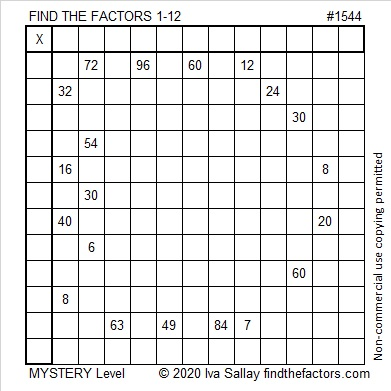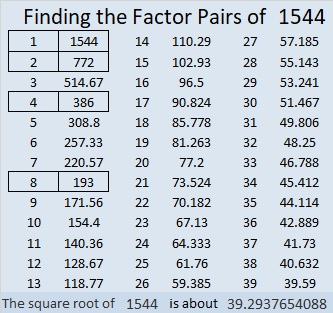# 1544 Final Letter of the Message

### Today’s Puzzle:

This is the sixth and final letter of the message that I made for you. It’s a mystery puzzle, but that doesn’t necessarily mean that’s it’s a difficult puzzle. Give it a try and think about why I sent the message.### Factors of 1544:

• 1544 is a composite number.
• Prime factorization: 1544 = 2 × 2 × 2 × 193, which can be written 1544 = 2³ × 193
• 1544 has at least one exponent greater than 1 in its prime factorization so √1544 can be simplified. Taking the factor pair from the factor pair table below with the largest square number factor, we get √1544 = (√4)(√386) = 2√386
• The exponents in the prime factorization are 3 and 1. Adding one to each exponent and multiplying we get (3 + 1)(1 + 1) = 4 × 2 = 8. Therefore 1544 has exactly 8 factors.
• The factors of 1544 are outlined with their factor pair partners in the graphic below.### More Facts about the Number 1544:

1544 is the sum of two squares:
38² + 10² = 1544.

1544 is the hypotenuse of a Pythagorean triple:
760-1344-1544, calculated from 2(38)(10), 38² – 10², 38² + 10².
It is also 8 times (95-168-193).